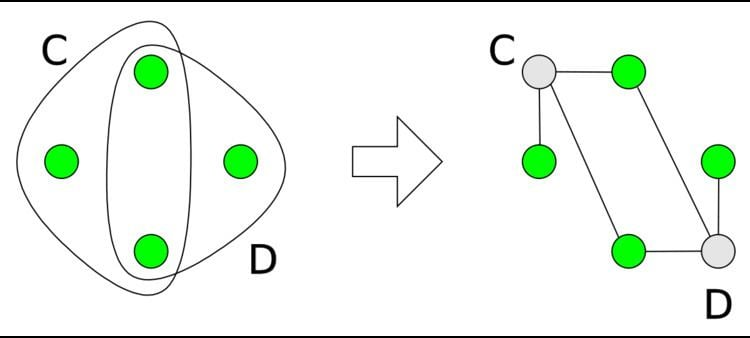# Hidden transformation

Updated onThe hidden transformation reformulates a constraint satisfaction problem in such a way all constraints have at most two variables. The new problem is satisfiable if and only if the original problem was, and solutions can be converted easily from one problem to the other.

There are a number of algorithms for constraint satisfaction that work only on constraints that have at most two variables. If a problem has constraints with a larger arity (number of variables), conversion into a problem made of binary constraints allows for execution of these solving algorithms. Constraints with one, two, or more variables are called unary, binary, or higher-order constraints. The number of variables in a constraint is called its arity.

The hidden transformation converts an arbitrary constraint satisfaction problem into a binary one. The transformation is similar to that generating the dual problem. The problem is added new variables, one for each constraint of the original problem. The domain of each such variable is the set of satisfying tuples of the corresponding constraint. The constraints of the new problem enforce the value of the original variables to be consistent with the values of the new variables. For example, if the new variables c , corresponding to the old constraint C ( x , y ) can assume values ( 1 , 2 ) and ( 2 , 0 ) , two new constraints are added: the first one enforces x to take value 1 if c = ( 1 , 2 ) value 2 if c = ( 2 , 0 ) , and vice versa. The second condition enforces a similar condition for variable y .

The graph representing the result of this transformation is bipartite, as all constraints are between a new and an old variable. Moreover, the constraints are functional: for any given value of a new variable, only one value of the old variable may satisfy the constraint.

## References

Similar Topics
Island of Lost Men
Abdul Malik Abdul Bashir
Neil Seery
Topics